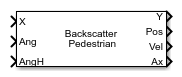# Backscatter Pedestrian

Backscatter signals from pedestrian

• Library:

•## Description

The Backscatter Pedestrian block models the monostatic reflection of non-polarized electromagnetic signals from a walking pedestrian. The pedestrian walking model coordinates the motion of 16 body segments to simulate natural motion. The model also simulates the radar reflectivity of each body segment. From this model, you can obtain the position and velocity of each segment and the total backscattered radiation as the body moves.

## Ports

### Input

expand all

Incident radar signals on each body segment, specified as a complex-valued M-by-16 matrix. M is the number of samples in the signal. See Body Segment Indices for the column representing the incident signal at each body segment.

The size of the first dimension of the input matrix can vary to simulate a changing signal length. A size change can occur, for example, in the case of a pulse waveform with variable pulse repetition frequency.

Data Types: `double`
Complex Number Support: Yes

Incident signal directions on the body segments, specified as a real-valued 2-by-16 matrix. Each column of `ANG` specifies the incident direction of the signal to the corresponding body part. Each column takes the form of an `[AzimuthAngle;ElevationAngle]` pair. Units are in degrees. See Body Segment Indices for the column representing the incident direction at each body segment.

Data Types: `double`

Heading of the pedestrian, specified as a scalar. Heading is measured in the xy-plane from the x-axis towards the y-axis. Units are in degrees.

Example: `-34`

Data Types: `double`

### Output

expand all

Combined reflected radar signals, returned as a complex-valued M-by-1 column vector. M equals the same number of samples as in the input signal, `X`.

Data Types: `double`
Complex Number Support: Yes

Positions of body segments, returned as a real-valued 3-by-16 matrix. Each column represents the Cartesian position, `[x;y;z]`, of one of 16 body segments. Units are in meters. See Body Segment Indices for the column representing the position of each body segment.

Data Types: `double`

Velocity of body segments, returned as a real-valued 3-by-16 matrix. Each column represents the Cartesian velocity, `[vx;vy;vz]`, of one of 16 body segments. Units are in meters per second. See Body Segment Indices for the column representing the velocity of each body segment.

Data Types: `double`

Orientation axes of body segments, returned as a real-valued 3-by-3-by-16 array. Each page represents the 3-by-3 orientation axes of one of 16 body segments. Units are dimensionless. See Body Segment Indices for the page representing the orientation of each body segment.

Data Types: `double`

## Parameters

expand all

Height of pedestrian, specified as a positive scalar. Units are in meters.

Data Types: `double`

Walking speed of the pedestrian, specified as a nonnegative scalar. The motion model limits the walking speed to 1.4 times the pedestrian height set in the Height (m) parameter. Units are in meters per second.

Data Types: `double`

Signal propagation speed, specified as a real-valued positive scalar. The default value of the speed of light is the value returned by `physconst('LightSpeed')`.

Data Types: `double`

Carrier frequency of narrowband incident signals, specified as a positive scalar. Units are in Hz.

Example: `1e9`

Data Types: `double`

Initial position of the pedestrian, specified as a 3-by-1 real-valued vector in the form of `[x;y;z]`. Units are in meters.

Data Types: `double`

Initial heading of the pedestrian, specified as a scalar. Heading is measured in the xy-plane from the x-axis towards y-axis. Units are in degrees.

Data Types: `double`

Block simulation, specified as `Interpreted Execution` or `Code Generation`. If you want your block to use the MATLAB® interpreter, choose `Interpreted Execution`. If you want your block to run as compiled code, choose ```Code Generation```. Compiled code requires time to compile but usually runs faster.

Interpreted execution is useful when you are developing and tuning a model. The block runs the underlying System object™ in MATLAB. You can change and execute your model quickly. When you are satisfied with your results, you can then run the block using ```Code Generation```. Long simulations run faster than in interpreted execution. You can run repeated executions without recompiling, but if you change any block parameters, then the block automatically recompiles before execution.

This table shows how the Simulate using parameter affects the overall simulation behavior.

When the Simulink® model is in `Accelerator` mode, the block mode specified using Simulate using overrides the simulation mode.

Acceleration Modes

 Block Simulation Simulation Behavior `Normal` `Accelerator` `Rapid Accelerator` `Interpreted Execution` The block executes using the MATLAB interpreter. The block executes using the MATLAB interpreter. Creates a standalone executable from the model. `Code Generation` The block is compiled. All blocks in the model are compiled.